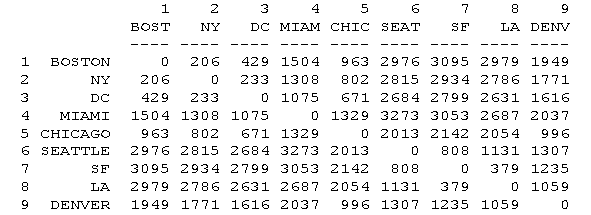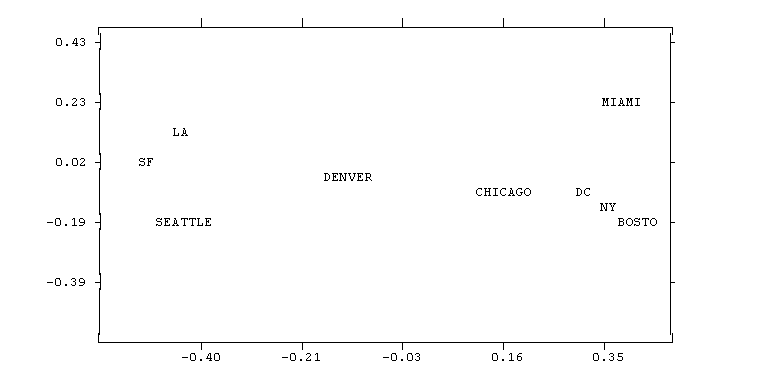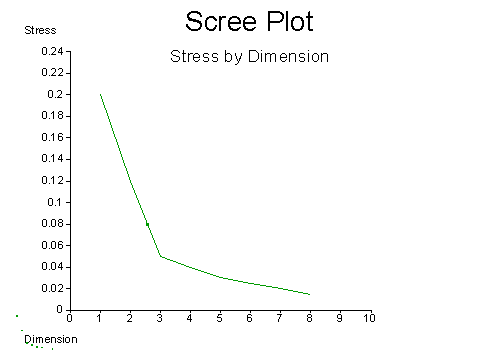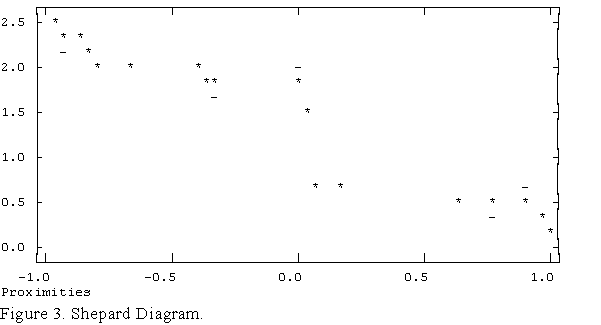Multidimensional Scaling

Overview

From a non-technical point of view, the purpose of multidimensional scaling (MDS) is to provide a visual representation of the pattern of proximities (i.e., similarities or distances) among a set of objects. For example, given a matrix of perceived similarities between various brands of air fresheners, MDS plots the brands on a map such that those brands that are perceived to be very similar to each other are placed near each other on the map, and those brands that are perceived to be very different from each other are placed far away from each other on the map.For instance, given the matrix of distances among cities shown above, MDS produces this map:In this example, the relationship between input proximities and distances among points on the map is positive: the smaller the input proximity, the closer (smaller) the distance between points, and vice versa. Had the input data been similarities, the relationship would have been negative: the smaller the input similarity between items, the farther apart in the picture they would be.

From a slightly more technical point of view, what MDS does is find a set of vectors in p-dimensional space such that the matrix of euclidean distances among them corresponds as closely as possible to some function of the input matrix according to a criterion function called stress.

A simplified view of the algorithm is as follows:

1. Assign points to arbitrary coordinates in p-dimensional space.
2. Compute euclidean distances among all pairs of points, to form the Dhat matrix.
3. Compare the Dhat matrix with the input D matrix by evaluating the stress function. The smaller the value, the greater the correspondance between the two.
4. Adjust coordinates of each point in the direction that best maximally stress.
5. Repeat steps 2 through 4 until stress won't get any lower.

Input Data

The input to MDS is a square, symmetric 1-mode matrix indicating relationships among a set of items. By convention, such matrices are categorized as either similarities or dissimilarities, which are opposite poles of the same continuum. A matrix is a similarity matrix if larger numbers indicate more similarity between items, rather than less. A matrix is a dissimilarity matrix if larger numbers indicate less similarity. The distinction is somewhat misleading, however, because similarity is not the only relationship among items that can be measured and analyzed using MDS. Hence, many input matrices are neither similarities nor dissimilarities.

However, the distinction is still used as a means of indicating whether larger numbers in the input data should mean that a given pair of items should be placed near each other on the map, or far apart. Calling the data "similarities" indicates a negative or descending relationship between input values and corresponding map distances, while calling the data "dissimilarities" or "distances" indicates a positive or ascending relationship.

A typical example of an input matrix is the aggregate proximity matrix derived from a pilesort task. Each cell xij of such a matrix records the number (or proportion) of respondents who placed items i and j into the same pile. It is assumed that the number of respondents placing two items into the same pile is an indicator of the degree to which they are similar. An MDS map of such data would put items close together which were often sorted into the same piles.

Another typical example of an input matrix is a matrix of correlations among variables. Treating these data as similarities (as one normally would), would cause the MDS program to put variables with high positive correlations near each other, and variables with strong negative correlations far apart.

Another type of input matrix is a flow matrix. For example, a dataset might consist of the number of business transactions occurring during a given period between a set of corporations. Running this data through MDS might reveal clusters of corporations that whose members trade more heavily with one another than other than with outsiders. Although technically neither similarities nor dissimilarities, these data should be classified as similarities in order to have companies who trade heavily with each other show up close to each other on the map.

Dimensionality

Normally, MDS is used to provide a visual representation of a complex set of relationships that can be scanned at a glance. Since maps on paper are two-dimensional objects, this translates technically to finding an optimal configuration of points in 2-dimensional space. However, the best possible configuration in two dimensions may be a very poor, highly distorted, representation of your data. If so, this will be reflected in a high stress value. When this happens, you have two choices: you can either abandon MDS as a method of representing your data, or you can increase the number of dimensions.

There are two difficulties with increasing the number of dimensions. The first is that even 3 dimensions are difficult to display on paper and are significantly more difficult to comprehend. Four or more dimensions render MDS virtually useless as a method of making complex data more accessible to the human mind. (However, there are other uses of MDS that are not affected by this problem.)

The second problem is that with increasing dimensions, you must estimate an increasing number of parameters to obtain a decreasing improvement in stress. The result is model of the data that is nearly as complex as the data itself.

On the other hand, there are some applications of MDS for which high dimensionality is not a problem. For instance, MDS can be viewed as a mathematical operation that converts an item-by-item matrix into an item-by-variable matrix. Suppose, for example, that you have a person-by-person matrix of similarities in attitudes. You would like to explain the pattern of similarities in terms of simple personal characteristics such as age, sex, income and education. The trouble is, these two kinds of data are not conformable. The person-by-person matrix in particular is not the sort of data you can use in a regression to predict age (or vice-versa). However, if you run the data through MDS (using very high dimensionality in order to achieve perfect stress), you can create a person-by-dimension matrix which is similar to the person-by-demographics matrix that you are trying to compare it to.

Stress

The degree of correspondence between the distances among points implied by MDS map and the matrix input by the user is measured (inversely) by a stress function. The general form of these functions is as follows:In the equation, dij refers to the euclidean distance, across all dimensions, between points i and j on the map, f(xij) is some function of the input data, and scale refers to a constant scaling factor, used to keep stress values between 0 and 1. When the MDS map perfectly reproduces the input data, f(xij) - dij is for all i and j, so stress is zero. Thus, the smaller the stress, the better the representation.

The stress function used in ANTHROPAC is variously called "Kruskal Stress", "Stress Formula 1" or just "Stress 1". The formula is:The transformation of the input values f(xij) used depends on whether metric or non-metric scaling. In metric scaling, f(xij) = xij. In other words, the raw input data is compared directly to the map distances (at least in the case of dissimilarities: see the section of metric scaling for information on similarities). In non-metric scaling, f(xij) is a weakly monotonic transformation of the input data that minimizes the stress function. The monotonic transformation is computed via "monotonic regression", also known as "isotonic regression".

From a mathematical standpoint, non-zero stress values occur for only one reason: insufficient dimensionality. That is, for any given dataset, it may be impossible to perfectly represent the input data in two or other small number of dimensions. On the other hand, any dataset can be perfectly represented using n-1 dimensions, where n is the number of items scaled. As the number of dimensions used goes up, the stress must either come down or stay the same. It can never go up.

Of course, it is not necessary that an MDS map have zero stress in order to be useful. A certain amount of distortion is tolerable. Different people have different standards regarding the amount of stress to tolerate. The rule of thumb we use is that anything under 0.1 is excellent and anything over 0.15 is unacceptable. Care must be exercised in interpreting any map that has non-zero stress since, by definition, non-zero stress means that some or all of the distances in the map are, to some degree, distortions of the input data. The distortions may be spread out over all pairwise relationships, or concentrated in just a few egregious pairs. In general, however, longer distances tend to be more accurate than shorter distances, so larger patterns are still visible even when stress is high. See the section on Shepard Diagrams and Interpretation for further information on this issue.

From a substantive standpoint, stress may be caused either by insufficient dimensionality, or by random measurement error. For example, a dataset consisting of distances between buildings in New York City, measured from the center of the roof, is clearly 3-dimensional. Hence we expect a 3-dimensional MDS configuration to have zero stress. In practice, however, there is measurement error such that a 3-dimensional solution does not have zero stress. In fact, it may be necessary to use 8 or 9 dimensions to bring stress down to zero. In this case, the fact that the "true" number of dimensions is known to be three allows us to use the stress of the 3-dimensional solution as a direct measure of measurement error. Unfortunately, in most datasets, it is not known in advance how many dimensions there "really" are.

In such cases we hope (with little foundation) that the true dimensionality of the data will be revealed to us by the rate of decline of stress as dimensionality increases. For example, in the distances between buildings example, we would expect significant reductions in stress as we move from a one to two to three dimensions, but then we expect the rate of change to slow as we continue to four, five and higher dimensions. This is because we believe that all further variation in the data beyond that accounted for by three dimensions is non-systematic noise which must be captured by a host of "specialized" dimensions each accounting for a tiny reduction in stress. Thus, if we plot stress by dimension, we expect the following sort of curve:Thus, we can theoretically use the "elbow" in the curve as a guide to the dimensionality of the data. In practice, however, such elbows are rarely obvious, and other, theoretical, criteria must be used to determine dimensionality.

Shepard Diagrams

The Shepard diagram is a scatterplot of input proximities (both xij and f(xij)) against output distances for every pair of items scaled. Normally, the X-axis corresponds to the input proximities and the Y-axis corresponds to both the MDS distances dij and the transformed ("fitted") input proximities f(xij). An example is given in Figure 3. In the plot, asterisks mark values of dij and dashes mark values of f(xij). Stress measures the vertical discrepancy between xij (the map distances) and f(xij) (the transformed data points). When the stress is zero, the asterisks and dashes lie on top of each other. In metric scaling, the asterisks form a straight line. In nonmetric scaling, the asterisks form a weakly monotonic function(1), the shape of which can sometimes be revealing (e.g., when map-distances are an exponential function of input proximities).If the input proximities are similarities, the points should form a loose line from top left to bottom right, as shown in Figure 3. If the proximities are dissimilarities, then the data should form a line from bottom left to top right. In the case of non-metric scaling, f(xij) is also plotted.

Interpretation

There are two important things to realize about an MDS map. The first is that the axes are, in themselves, meaningless and the second is that the orientation of the picture is arbitrary. Thus an MDS representation of distances between US cities need not be oriented such that north is up and east is right. In fact, north might be diagonally down to the left and east diagonally up to the left. All that matters in an MDS map is which point is close to which others.

When looking at a map that has non-zero stress, you must keep in mind that the distances among items are imperfect, distorted, representations of the relationships given by your data. The greater the stress, the greater the distortion. In general, however, you can rely on the larger distances as being accurate. This is because the stress function accentuates discrepancies in the larger distances, and the MDS program therefore tries harder to get these right.

There are two things to look for in interpreting an MDS picture: clusters and dimensions. Clusters are groups of items that are closer to each other than to other items. For example, in an MDS map of perceived similarities among animals, it is typical to find (among north americans) that the barnyard animals such as chicken, cow, horse, and pig are all very near each other, forming a cluster. Similarly, the zoo animals like lion, tiger, antelope, monkey, elephant and giraffe form a cluster. When really tight, highly separated clusters occur in perceptual data, it may suggest that each cluster is a domain or subdomain which should be analyzed individually. It is especially important to realize that any relationships observed within such a cluster, such as item a being slightly closer to item b than to c should not be trusted because the exact placement of items within a tight cluster has little effect on overall stress and so may be quite arbitrary. Consequently, it makes sense to extract the submatrix corresponding to a given cluster and re-run the MDS on the submatrix.(2) (In some cases, however, you will want to re-run the data collection instead.)

Dimensions are item attributes that seem to order the items in the map along a continuum. For example, an MDS of perceived similarities among breeds of dogs may show a distinct ordering of dogs by size. The ordering might go from right to left, top to bottom, or move diagonally at any angle across the map. At the same time, an independent ordering of dogs according to viciousness might be observed. This ordering might be perpendicular to the size dimension, or it might cut a sharper angle.

The underlying dimensions are thought to "explain" the perceived similarity between items. For example, in the case of similarities among dogs we expect that the reason why two dogs are seen as similar is because they have locations or scores on the identified dimensions. Hence, the observed similarity between a doberman and a german shepherd is explained by the fact that they are seen as nearly equally vicious and about the same size. Thus, the implicit model of how similarity judgments are produced by the brain is that items have attributes (such as size, viciousness, intelligence, furriness, etc) in varying degrees, and the similarity between items is a function of their similarity in scores across all attributes. This function is often conceived of as a weighted sum of the similarity across each attribute, where the weights reflect the importance or saliency of the attribute.

It is important to realize that these substantive dimensions or attributes need not correspond in number or direction to the mathematical dimensions (axes) that define the vector space (MDS map). For example, the number of dimensions used by respondents to generate similarities may be much larger than the number of mathematical dimensions needed to reproduce the observed pattern. This is because the mathematical dimensions are necessarily orthogonal (perpendicular), and therefore maximally efficient. In contrast, the human dimensions, while cognitively distinct, may be highly intercorrelated and therefore contain some redundant information.

One thing to keep in mind in looking for dimensions is that your respondents may not have the same views that you do. For one thing, they may be reacting to attributes you have not thought of. For another, even when you are both using the same set of attributes, they may assign different scores on each attribute than you do. For example, one of the attributes might be "attractiveness". Your view of what an attractive dog, person, fruit or other item may be very different from your respondents'.(3)

Useful References

Methodological

• Kruskal, J.B. and M. Wish 1978. Multidimensional Scaling. Sage.

Applications

• Moore, Rod 1990. "Ethnographic assessment of pain coping perceptions." Psychosomatic Medicine 52:171-181.
• Blank & Mattes "Sugar and Spice: Similarities and Sensory Attributes" Nursing Research 39(5):290-293
• Wexler & Romney. 1972. "Individual Variations in cognitive structures." in Romney, Shepard & Nerlove eds. Multidimensional Scaling: Theory and Applications in the behavioral sciences, Vol II. Seminar Press.

### End Notes:

1. If the input data dissimilarities, the function is never decreasing. If the input data are similarities, the function is never increasing.

2. In some cases, however, it is better to rerun the data collection on the subset of items. This is because the presence of the other items can evoke additional dimensions/attributes of comparison that could affect the way items in the subset are viewed.

3. Fortunately, a very simple technique exists to deal with this problem. The technique is called property fitting (PROFIT).

[geneva97/eop.htm]